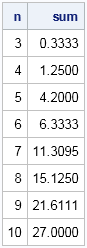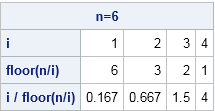How do we go about summing a finite series in SAS? For example, I want to computefor various integers n ≥ 3. I want to output two columns, one for the natural numbers and one for the summation of the series.

Summations arise often in statistical computing, so this is a great question. I don't understand why the upper limit only goes to n–2, but I think you'll see that the programs below can compute sums for any upper limit.

You can compute summations in the DATA step by using a DO loop. To compute them efficiently in the SAS/IML language, you usually want to construct a vector such that the ith element of the vector is the ith term of the series. Then you use the SUM function to add the terms of the series.

### A summation by using the DATA step

It is easy to sum a series by using the DATA step. You set the value of the sum to 0, then loop over the values of i, summing up each term as you go. For this example, the ith term is i / floor(n/i), and the summation is over the terms i=1 to i=n–2. The following DATA step computes this summation for a sequence of values of n:

```data Series; do n = 3 to 10; sum = 0; do i = 1 to n-2; sum = sum + i/ floor(n/i); end; output; end; keep n sum; run;   proc print data=Series noobs; run;```### A vectorized summation

In a vector language such as R, MATLAB, or SAS/IML, an efficient summation begins by constructing vectors that contain the elements that are being summed. Suppose that you define i to be a vector that contains the sequence 1, 2, ..., n–2. Then the expression floor(n/i) is also a vector, as is the elementwise ratio of these vectors. These three vectors are shown in the rows of the following table:The bottom row of the table contains the terms of the series. Notice that the terms sum to 6.333, which agrees with the output from the DATA step in the previous section. Use the SUM function to add the terms, as shown in the following function, which computes the summation Sn for an arbitrary value of n > 2:

```proc iml; start SumSeries(n); i = 1:(n-2); /* index of terms */ return( sum(i / floor(n/i)) ); /* sum of terms */ finish;```

If you want the summation for several values of n, you can use a DO loop to iterate over values of n. The result is the same as for the DATA step program.

```n = T(3:10); sum = j(nrow(n),1); /* allocate a vector for the results */ do k = 1 to nrow(n); sum[k] = SumSeries( n[k] ); end; print n sum;```

### A matrix approach to summation

The previous section shows an efficient way to use vectorized operations to compute a summation. However, just for fun, let's see if we can compute the summation for MANY values of n without writing ANY loops! As is typical, a matrix approach uses more memory.

The main idea is to construct a lower triangular matrix whose nth row contains the terms for Sn. You can start by constructing the lower triangular matrix A whose rows contains the vector 1:(n–2) for various values of n. In order to avoid dividing by 0 when you form the expression floor(n/i), you should use missing values for the upper triangular elements of the matrix. The ROW and COL functions are useful for constructing the matrix, as shown below. The ROW and COL functions were introduced in SAS/IML 12.3, but if you are running an earlier version of SAS you can find the definitions in a previous blog post.

```nMax = 10; A = col( j(nMax-2, nMax-2) ); /* each row is {1 2 3 ... 10} */ A[loc(col(A)>row(A))] = .; /* set upper triangular elements to missing */ *print A;```

If you define the column vector n = {3, 4, ..., 10}, then each row of the matrix floor(n/A) contains the denominators for the series. To compute the summation for all values of n, you can form the expression A / floor(n/A) and compute the sums across each row by using the summation subscript reduction operator, as follows:

```n = T(3:nMax); /* column vector */ B = A / floor( n/A ); /* each row contains terms of series for a given n value */ sum = B[,+]; /* sum across rows */ print n sum;```

Although I wouldn't ordinarily use the matrix method to sum a series, the technique is useful for constructing structured matrices whose elements are given by a formula. A canonical example is the Hilbert matrix.

ShareDistinguished Researcher in Computational Statistics

Rick Wicklin, PhD, is a distinguished researcher in computational statistics at SAS and is a principal developer of SAS/IML software. His areas of expertise include computational statistics, simulation, statistical graphics, and modern methods in statistical data analysis. Rick is author of the books Statistical Programming with SAS/IML Software and Simulating Data with SAS.

1.Dear Rick

Thank you so much for your useful blog. I have a question regrading finding the summation. I have two summation: the first one is ( n=0 to 5) and the second one is (i=0 to 2*n). I'm totally confused how to write these two summation.

Thank You
Alex

•You need to specify the "body" of the summation as well as the language (DATA step? IML?). I think it would be easier to answer your questio if you ask it at the SAS Support Communities.

2.Hello Rick,

I have a question regarding recursive/cumalitive addition of a parrticular column for exampe:

A Sum(A)
1 . 1
2 . 1+2=3
3 1+2+3=6

and so on

•```data Have; do A = 1 to 10; output; end; run;   data Want; set Have; cusum + A; /* equivalent to RETAIN cusum; cusum=SUM(cusum,A); */ run;```